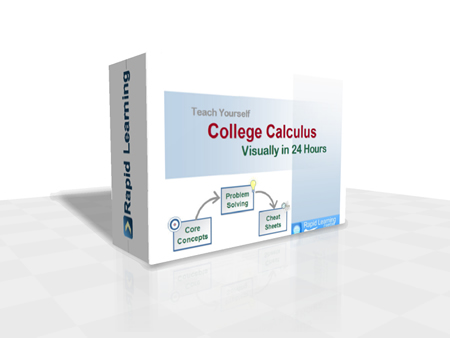How to Learn in 24 Hours?

 Need Help? M-F: 9am-5pm(PST): Toll-Free: (877) RAPID-10 or 1-877-727-4310 24/7 Online Technical Support: The Rapid Support Center vip@rapidlearningcenter.com Secure Online Order:Need Proof? Testimonials by Our Users

 Rapid Learning Courses: MCAT in 24 Hours (2021-22) USMLE in 24 Hours (Boards) Chemistry in 24 Hours Biology in 24 Hours Physics in 24 Hours Mathematics in 24 Hours Psychology in 24 Hours SAT in 24 Hours ACT in 24 Hours AP in 24 Hours CLEP in 24 Hours DAT in 24 Hours (Dental) OAT in 24 Hours (Optometry) PCAT in 24 Hours (Pharmacy) Nursing Entrance Exams Certification in 24 Hours eBook - Survival Kits Audiobooks (MP3)

 Tell-A-Friend: Have friends taking science and math courses too? Tell them about our rapid learning system.Home »  Mathematics »  College CalculusCourse Preview

This college calculus rapid learning series is designed for college students who are required to take a single calculus course, typically for non-math/engineering majors.

This comprehensive course includes precalculus review, limits, derivatives and integrals, with the focus on conceptual understanding and problem solving. The visual tutorials with expert narration provide the non-intimidating introduction to the often-challenging topics, making calculus learning fun and easy.

1. 24 Core Concept Tutorials (Flash Movies)
24x visual tutorials for 24 chapters, with concept map, concept illustration, step-wise problem solving and topical summary, narrated by college calculus professors.
2. 24 Problem-Solving Drills (Flash Games)
24x feedback based Quizzes, concept-based problems, summary reviews on all problems and scoring system to track performance.
3. 24 Super Review Cheat Sheets (PDF Printables)
One cheat sheet per topic, key concepts in a single-sheet, at-a-glance review of each topic and both printable and laminatible Ideal for exam prep quick review.
Pre-requisites for Calculus: (1) Elementary Algebra - RL409 (2) Intermediate Algebra - RL407 (3) Pre-Calculus - RL403.

College Calculus Rapid Learning Series

This 24-chapter series provides comprehensive coverage of traditional college calculus topics for any students who are taking business calculus and calculus for life science, social science and other non-math majors.

Core Unit #1 – The Foundation

• Tutorial 01: Introduction to Calculus
• Tutorial 02: Precalculus Review

Core Unit #2 – Functions and Graphs

• Tutorial 03: Functions and Graphs
• Tutorial 04: Exponential Functions
• Tutorial 05: Inverse Functions and Logarithms

Core Unit #3 – Limits and Continuity

• Tutorial 06: Limits
• Tutorial 07: Trigonometric Limits and Continuity
• Tutorial 08: Limits Involving Infinity
• Tutorial 09: Rates of Changes

Core Unit #4 – Derivatives

• Tutorial 10: The Derivative and the Tangent Line
• Tutorial 11: Differentiability and Rules of Differentiation
• Tutorial 12: The Chain Rule and High Derivatives
• Tutorial 13: Implicit Differentiation and Related Rates
• Tutorial 14: Applications of Differentiation - Part 1
• Tutorial 15: Applications of Differentiation - Part 2

Core Unit #5 – Integrals

• Tutorial 16: Riemann Sums and Definite Integrals
• Tutorial 17: The Fundamental Theorem of Calculus
• Tutorial 18: Antidifferentiation and Integration by Substitution
• Tutorial 19: Numerical Integration
• Tutorial 20: Applications of Integration
• Tutorial 21: Differential Equations and Mathematical Modeling

Core Unit #6 – Critical Skills

• Tutorial 22: Problem Solving in Calculus
• Tutorial 23: Graphing Utilities in Calculus
• Tutorial 24: Calculus Exam Guide

Master Calculus the rich-media way, get started now:

Course Preview